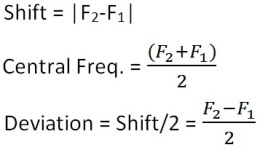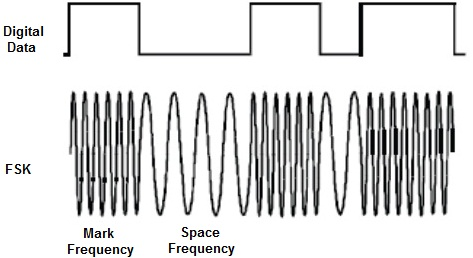Demodulation of Frequency Shift Keying Signal

Objective

To study Demodulation of Frequency Shift Keying Signal (FSK) signal using filtering and an envelope detector.

Introduction

We use FSK modulator for long distance communication, which the voltage level of digital signal has been converted to frequency. Therefore, at the receiver, we have to recover the FSK signal to digital signal that means the frequency should be converted back to voltage. We use phase locked loop (PLL) as FSK demodulator. PLL is a kind of automatic tracking system, which is able to detect the input signal frequency and phase. PLL is widely used in wireless applications, such as AM demodulator, FM demodulator, and frequency selector and so on. In the digital communications, various types of digital PLLs are developed. Digital PLL is very useful in carrier synchronization, bit synchronization and digital demodulation.

Frequency-shift keying is a type of digital binary communication technique. It is identical to FM modulation using a digital binary signal as the message m(t). Thus, a binary 1 represents one frequency, and a binary 0 represents another frequency. The FSK signal deviates from the carrier frequency depending on the binary message m(t). For example, assume m(t) can take on the values 1 or -1. When m(t) = 1, the FSK signal would deviate on the lower side of the carrier frequency. When m(t) = -1, the FSK signal would deviate to the higher side of the carrier frequency. The frequency deviation of the FSK signal is given by the FM equation:In this example, Vp = 1 & -1. So, the deviation of the FSK signal is ± ( Df ) / 2π about the carrier. The modulation parameter Df is chosen to give the desired amount of deviation in the FSK modulated signal. The deviation, shift & central frequency can be calculated asFig.1 FSK Modulation.